Next: Worked example 11.5: Gravity Up: Oscillatory motion Previous: Worked example 11.3: Block

## Worked example 11.4: Energy in simple harmonic motion

Question: A block of mass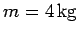is attached to a spring, and undergoes simple harmonic motion with a period of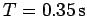. The total energy of the system is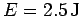. What is the force constant of the spring? What is the amplitude of the motion?

Answer: The angular frequency of the motion is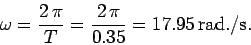Now,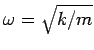for a mass on a spring. Rearrangement of this formula yields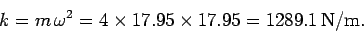The total energy of a system executing simple harmonic motion is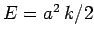. Rearrangement of this formula gives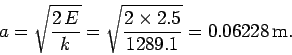Thus, the force constant is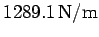and the amplitude is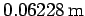.

Richard Fitzpatrick 2006-02-02